The goal of lcmr is to simulate phenomena of classical conditioning and fit the conditioning data with the latent cause model.

lcmr is an R package of Latent Cause Model: LCM and Latent Cause Modulated Rescorla-Wagner model: LCM-RW made bySam Gershman.

## Installation

You can install the development version from GitHub with:

# install.packages("devtools")
devtools::install_github("ykunisato/lcmr")

## List of functions

• fit_lcm: Fit model to conditioning data
• infer_lcm: Simulate the classical conditioning with LCM
• infer_lcm_rw: Simulate the classical conditioning with LCM-RW

## How to simulate the renewal effect with LCM

The renewal effect is the phenomenon in which the change of context after extinction can lead to a return of conditioned response (CR). infer_lcm(X,opts) can simulate the renewal effect.

First, you have to prepare the design of conditioning. In followings, I prepare the experimental design consisted from acquisition (CS presents with US in context A(context = 1), 10 trials), extinction (CS presents without US in context B(context = 0),10 trials) and test (CS without US in context A(context = 0), 10 trials).

US <-      c(1,1,1,1,1,1,1,1,1,1,  0,0,0,0,0,0,0,0,0,0, 0,0,0,0,0,0,0,0,0,0)
CS <-      c(1,1,1,1,1,1,1,1,1,1,  1,1,1,1,1,1,1,1,1,1, 1,1,1,1,1,1,1,1,1,1)
Context <- c(1,1,1,1,1,1,1,1,1,1,  0,0,0,0,0,0,0,0,0,0, 1,1,1,1,1,1,1,1,1,1)
X <- cbind(US, CS, Context)

You can simulate the conditioned response using infer_lcm(). I simulate the conditioned response setting the alpha = 0.4 . The alpha is the concentrate parameter of the Chinese Restaurant Process.

library(lcmr)
sim_res <- infer_lcm(X = X, opts = list(c_alpha = 1))

You can the plot of change of conditioned response through the trials using the following codes.

library(tidyverse)
sim_data <- data.frame(X, Trial = seq(1,length(US)), CR = sim_res$V) sim_data %>% ggplot(aes(x = Trial, y = CR)) + geom_line() + ylim(0,1)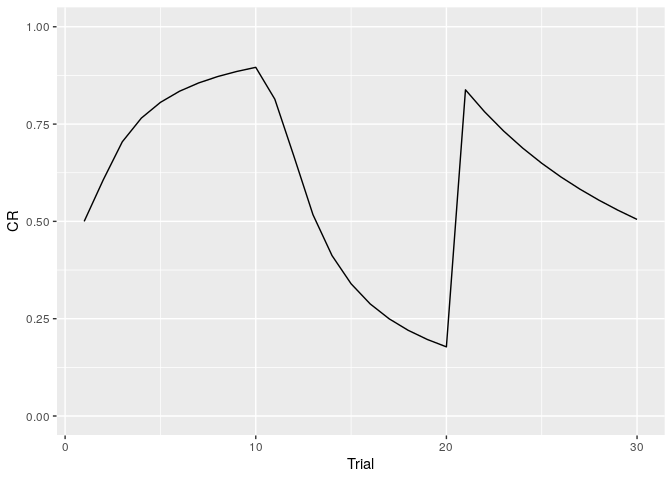You can draw the plot of the posterior probability of each cause using the following codes. sim_post <- as.data.frame(sim_res$post)
sim_post %>%
mutate(Trial = seq(1,length(US))) %>%
rename(C01 = V1, C02 = V2, C03 = V3, C04 = V4, C05 = V5,
C06 = V6, C07 = V7, C08 = V8, C09 = V9, C10 = V10) %>%
gather(key = "Cause", value = "post",-Trial) %>%
mutate(Cause = as.factor(Cause)) %>%
ggplot(aes(x = Trial, y = post, color = Cause)) +
geom_line() +
ylim(0,1) +
labs(y="Posterior probability")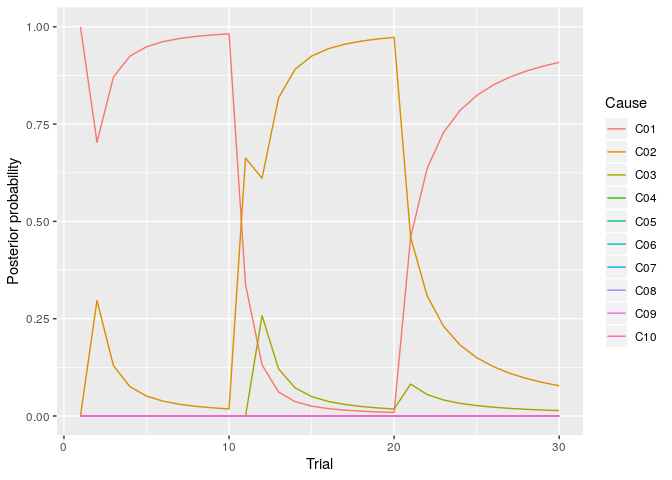## How to simulate the spontaneous recovery with LCM-RW

The spontaneous recovery is the phenomenon in which the time elapses following extinction can lead to a return of conditioned response (CR). infer_lcm_rw(X,opts) can simulate the spontaneous recovery.

First, you have to prepare the design of conditioning. In followings, I prepare the experimental design consisted from acquisition (CS1 presents with US, CS2 presents without US, 9 trials each CS), extinction (both CSs presents without US, 6 trials each CS) and test (both CSs presents without US after 1 day, 3 trials each CS).

US  <- c(1,0,0,1,1,0,1,0,1,1,0,0,1,0,0,1,1,0, 0,0,0,0,0,0,0,0,0,0,0,0, 0,0,0,0,0,0)
CS1 <- c(1,0,0,1,1,0,1,0,1,1,0,0,1,0,0,1,1,0, 1,1,0,1,0,0,1,0,1,1,0,0, 1,0,0,1,1,0)
CS2 <- c(0,1,1,0,0,1,0,1,0,0,1,1,0,1,1,0,0,1, 0,0,1,0,1,1,0,1,0,0,1,1, 0,1,1,0,0,1)
time <- c(0, seq(1:17)*4, 100+seq(1:12)*4, 86400+seq(1:6)*4)
X <- cbind(time, US, CS1, CS2)

You can simulate the conditioned response using infer_lcm(). I simulate the conditioned response setting the alpha = 0.45 and the eta = 0.2. The alpha is the concentrate parameter of the Chinese Restaurant Process and eta is the learning rate of the RW model.

library(lcmr)
sim_res <- infer_lcm_rw(X = X, opts = list(c_alpha = 0.45, eta = 0.2))

You can the plot of change of conditioned response through the trials using the following codes.

library(tidyverse)
sim_data <- data.frame(X,
Trials_cs1 = cumsum(CS1),
Trials_cs2 = cumsum(CS2),
CR = sim_res$V) sim_data %>% filter(CS1 == 1) %>% ggplot(aes(x = Trials_cs1, y = CR)) + geom_line() + ylim(0,1) + labs(x = "Trial", y = "CR of CS1")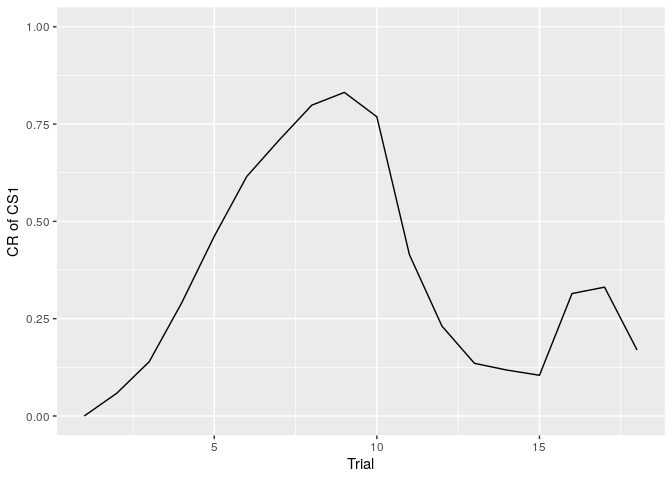sim_data %>% filter(CS2 == 1) %>% ggplot(aes(x = Trials_cs2, y = CR)) + geom_line() + ylim(0,1) + labs(x = "Trial", y = "CR of CS2")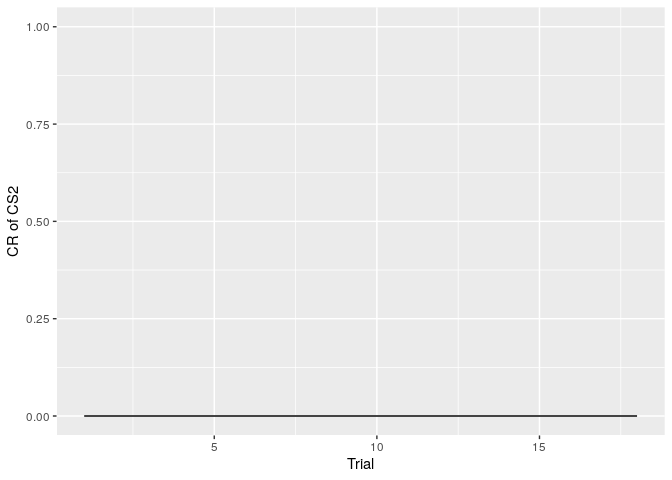## How to fit LCM to the conditioning data. You have to prepare the data as long format containing the following variables (Order and name is exactly the same as following): • ID Subject ID • CR Conditioned Response • US Unconditioned Stimulus • CS Conditioned Stimului or Context. If using multiple CS, set variables name as CS1,CS2,CS3… I make the synthetic data for model fitting with the experiment design of the renewal effect. US <- c(1,1,1,1,1,1,1,1,1,1, 0,0,0,0,0,0,0,0,0,0, 0,0,0,0,0,0,0,0,0,0) CS <- c(1,1,1,1,1,1,1,1,1,1, 1,1,1,1,1,1,1,1,1,1, 1,1,1,1,1,1,1,1,1,1) Context <- c(1,1,1,1,1,1,1,1,1,1, 0,0,0,0,0,0,0,0,0,0, 1,1,1,1,1,1,1,1,1,1) X <- cbind(US, CS, Context) I make synthetic data for 100 participants. number_of_perticiapnts <- 100 participants_alpha <- runif(number_of_perticiapnts, 0, 10) data <- NULL for (i in 1:number_of_perticiapnts) { sim_data <- infer_lcm(X, opts = list(c_alpha = participants_alpha[i], M = 1000)) sim_df <- data.frame(ID = rep(i,length(US)), CR = sim_data$V, X)
data <- rbind(data,sim_df)
}

You can estimate parameter alpha using fit_lcm(data, model, opts, parameter_range, parallel, estimation_method). You have to specify the following argument for model fitting:

• model: 1 = latent cause model, 2 = latent cause modulated RW model

• parameter_range: range of parameter(a_L, a_U, e_L, e_U)

• stimation_method: 0 = optim or optimize(lcm), 1 = post mean(only latent cause model)

results <- fit_lcm(data,
model = 1,
parameter_range = list(a_L = 0, a_U = 15),
estimation_method = 0)

I check the parameter recovery. It looks well recovery of parameter alpha.

parameter_recovery <- data.frame(true_alpha = participants_alpha, estimated_alpha = results$parameters$alpha)
parameter_recovery %>%
ggplot(aes(x = true_alpha, y= estimated_alpha)) +
geom_point()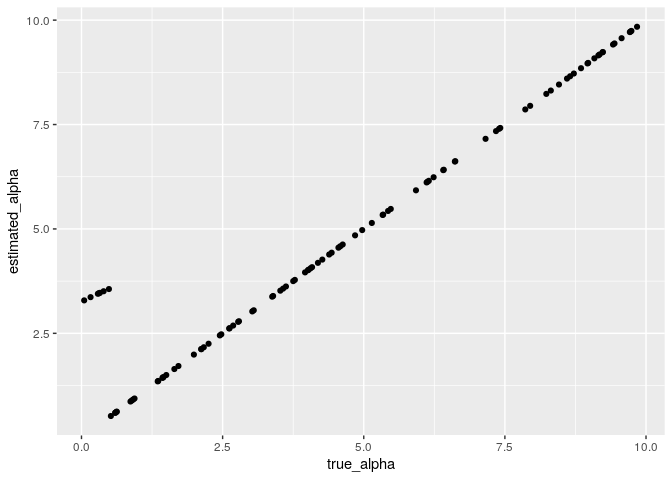## How to fit LCM-RW to the conditioning data.

The fit_lcm() allow to estimate alpha and eta of LCM-RW (set model = 2). However, fit_lcm() can not to estimate parameter adequatly at this time(fit_lcm can not recover the parameter adequately).

## Bugs and question

Please report on this repository’s issues